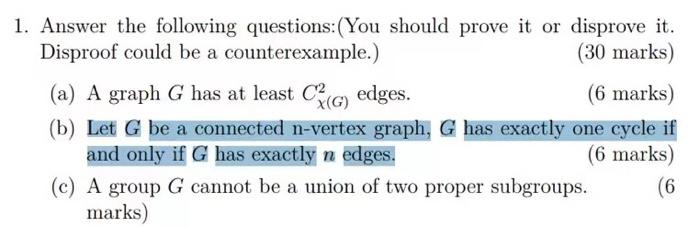# (Solved): 1. Answer the following questions:(You should prove it or disprove it. Disproof could be a countere ...1. Answer the following questions:(You should prove it or disprove it. Disproof could be a counterexample.) (30 marks) (a) A graph $$G$$ has at least $$C_{\chi(G)}^{2}$$ edges. (6 marks) (b) Let $$G$$ be a connected n-vertex graph, $$G$$ has exactly one cycle if and only if $$G$$ has exactly $$n$$ edges. (6 marks) (c) A group $$G$$ cannot be a union of two proper subgroups. $$(6$$ marks)

We have an Answer from Expert